Get inspired by the success stories of our students in IIT JAM MS, ISI  MStat, CMI MSc DS.  Learn More

# ISI MStat 2015 PSA Problem 18 | Complex NumberThis is a beautiful problem from ISI MSTAT 2015 PSA problem 18 based on complex number. We provide sequential hints so that you can try.

## Complex Number - ISI MStat Year 2015 PSA Question 18

The set of complex numbers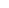satisfying the equation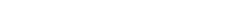represents, in the complex plane

• a straight line
• a pair of intersecting straight lines
• a point
• a pair of distinct parallel straight lines

### Key Concepts

Complex number representation

Straight line

Answer: is a pair of intersecting straight lines

ISI MStat 2015 PSA Problem 18

Precollege Mathematics

## Try with Hints

Simplify the Complex. Just Solve.

Let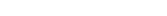Then the given equation reduces to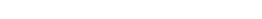.
Which implies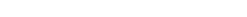.
They do intersect.(?)

Yes! they intersect and to get the point of intersection just use substitution . Hence it gives a pair of intersecting straight lines.

## Subscribe to Cheenta at Youtube

This is a beautiful problem from ISI MSTAT 2015 PSA problem 18 based on complex number. We provide sequential hints so that you can try.

## Complex Number - ISI MStat Year 2015 PSA Question 18

The set of complex numberssatisfying the equationrepresents, in the complex plane

• a straight line
• a pair of intersecting straight lines
• a point
• a pair of distinct parallel straight lines

### Key Concepts

Complex number representation

Straight line

Answer: is a pair of intersecting straight lines

ISI MStat 2015 PSA Problem 18

Precollege Mathematics

## Try with Hints

Simplify the Complex. Just Solve.

LetThen the given equation reduces to.
Which implies.
They do intersect.(?)

Yes! they intersect and to get the point of intersection just use substitution . Hence it gives a pair of intersecting straight lines.

## Subscribe to Cheenta at Youtube

This site uses Akismet to reduce spam. Learn how your comment data is processed.

### Knowledge Partner